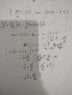# Indefinite integral of cross product of 2 function

• agnimusayoti

#### agnimusayoti

Homework Statement
If ##\vec{v} (t)## is a vector function of t, find indefinite integral
$$\int {\left(\vec v \times \frac{d^2 \vec v}{dt^2} dt\right)}$$
Relevant Equations
Idk.
I've tried with this work in attachment. i&m not sure of my answer is correct.

#### Attachments

•15926584704436099750539116260485.jpg
36.3 KB · Views: 150
The problem statement is not given properly. Can you provide us proper details? If you are computing:

$$\int v \frac{d^2v}{dt^2} dt$$​

You mentioned ##dv = b(t) dt##, and then computed ##v##:

$$v = \frac{dv}{dt}$$​

You know this is inherently not correct, unless the function is specifically ##v(t) = e^t##. Don't forget about generality when writing math proofs, or computing such things.

•Delta2
The problem statement is not given properly. Can you provide us proper details? If you are computing:
$$\int v \frac{d^2v}{dt^2} dt$$​
The thread title says cross product.

Last edited:
@agnimusayoti , I think you confused everyone with your unfortunate choice of notation, using v (no arrow) for ##\frac{d\vec v}{dt}## and u for ##\vec v##.
Where your algebra goes wrong is in handling ##\int \frac{d\vec v}{dt}\times d\vec v##. The correct result becomes obvious if you write it as ##\int \frac{d\vec v}{dt}\times \frac{d\vec v}{dt}dt##